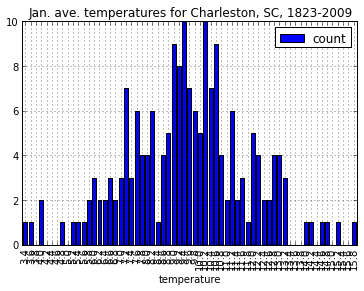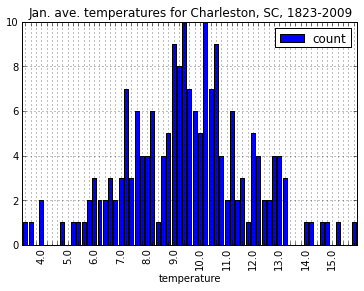### Solving x axis overprinting on Pandas/Matplotlib bar charts

If creating a histogram based on "binning" a continuous variable such as temperature or distance, it would be inappropriate to use a line chart since the y-axis "counts of occurrences" would be meaningless without representing the bin width.

First, we grab some temperature data, compute a histogram, and store the histogram data into a new data frame called dfh.

In :
from pandas import *
h=histogram(df.ix[map(lambda x: x.strip()=="CHARLESTON",df["Station"]),6],bins=arange(3.4,16.2,0.2))
dfh = DataFrame(zip(h,h),columns=["temperature","count"])


A straightforward plot() of dfh results in overprinted x axis labels.

Note: this example is somewhat contrived in that we could have just called df.hist() (on the original un-histogrammed data) instead of dfh.plot(), and Pandas/Matplotlib does a fine job when plotting with hist(). So the assumption where this is relevant is that the data you have has already been histogrammed and the original un-histogrammed data is unavailable to you.

In :
dfh.plot(kind="bar",x="temperature",title="Jan. ave. temperatures for Charleston, SC, 1823-2009")

Out:
<matplotlib.axes.AxesSubplot at 0x109d5ab50>The solution is to call set_xticklabels and give it a list of the labels we want, putting in "" for the labels we want to skip. The code below achieves it in a single line of code.

In :
ax=dfh.plot(kind="bar",x="temperature",title="Jan. ave. temperatures for Charleston, SC, 1823-2009")
r=ax.set_xticklabels(map(lambda x: 3.4+x/5.0 if (x+2)%5==0 else "", range(65)))In :